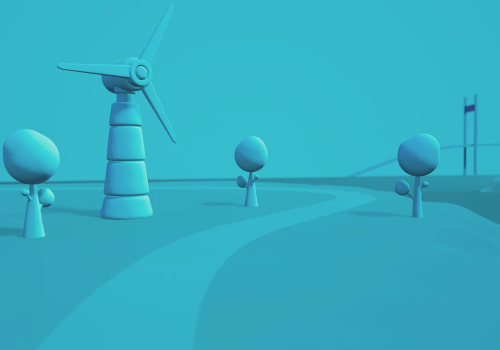# Frank Energie

Energie against dynamic tarifs## Flow cards

#### When...

Frank Energie
Electricity price changed
Frank Energie
Electricity price is Percentage higher than the day average
Frank Energie
Electricity price is Percentage higher than the next Hours hours
Frank Energie
Electricity price is higher than the next Hours hours
Frank Energie
Electricity price is the highest of the day
Frank Energie
Electricity price is the lowest of the day
Frank Energie
Electricity price is Percentage lower than the day average
Frank Energie
Electricity price is Percentage lower than the next Hours hours
Frank Energie
Electricity price is lower than the next Hours hours
Frank Energie
Gas price changed

#### And...

Frank Energie
Electricity price is Percentage higher than the day average
Frank Energie
Electricity price is Percentage higher than the next Hours hours
Frank Energie
Electricity price is higher than the next Hours hours
Frank Energie
Electricity price is higher than Price
Frank Energie
Electricity price is the highest of the day
Frank Energie
Electricity price is lower than Price
Frank Energie
Electricity price is the lowest of the day
Frank Energie
Electricity price is Percentage lower than the day average
Frank Energie
Electricity price is Percentage lower than the next Hours hours
Frank Energie
Electricity price is lower than the next Hours hours
Frank Energie
Gas price is higher than Price
Frank Energie
Gas price is lower than Price

## Reviews

This app has no reviews yet.

You can leave a review once you're using this app.## Support

Having an issue with this app? Contact the developer by sending an e-mail.

## What's newFrank Energie is not compatible with the selected Homey.Frank Energie will install on Homey shortly.